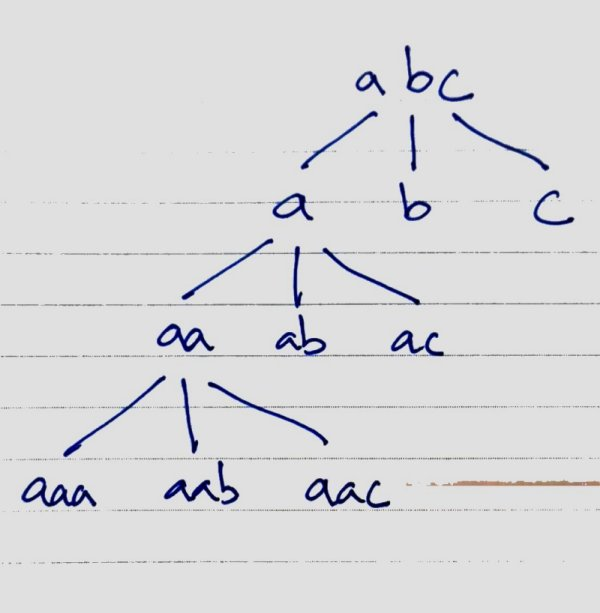# Permutations With Repeating Characters (Python)

February 22, 2019

Generate permutations of `n` elements (including repeating/duplicate elements)

``````ab = aa, bb, ab, ba
abc = aaa, aab, abc ...
``````

The number of combination should be `n^k` (n=number of elements, k=combination length). For this case, `n` and `k` happens to be the same.

``````ab = 2^2 = 4
abc = 3^3 = 9

If we use `abc`(n=3) to generate permutations of length 1 (k=1)
P(abc, 1) = a, b, c = 3^1 = 3 combinations

If we use `abc`(n=3) to generate permutations of length 2 (k=2)
P(abc, 2) = aa, bb, cc, ab, ac ... = 3^2 = 9 combinations
``````

Linear iteration of all elements is unlikely to generate all combinations, so a recursive solution is more likely.

Imagine the following diagram.Result of P(a) is used to generate P(ab). We start with a prefix of 1 character, then we more character to prefix until all combination are generated recursively.

``````def _permutation_repeat(text, prefix, n, k):
if k == 0: # base, len(prefix) == len(text)
print(prefix)
return

for i in range(n):
new_prefix = prefix + text[i] # a, aa, aaa, aab, aac ab, aba, abb, abc

# print(new_prefix)
_permutation_repeat(text, new_prefix, n, k-1)
# print('---')

def permutation_repeat(text, k):
_permutation_repeat(text, "", len(text), k)

permutation_repeat("abc", 3)
``````

The combination is generated in the following sequence.

``````a ->
aa ->
aaa
aab
aac
ab ->
aba
abb
abc
ac ->
aca
acb
acc
b ->
ba ->
baa
bab
bac
...
...
``````

Output

``````aaa
aab
aac
aba
abb
abc
aca
acb
acc
baa
bab
bac
bba
bbb
bbc
bca
bcb
bcc
caa
cab
cac
cba
cbb
cbc
cca
ccb
ccc
``````

Permutation with repeats in result is actually Cartesian Product.

You can use Python itertools.product to generate the same result.

``````from itertools import product

for _set in product(list('abc'), repeat=3):
print(''.join(_set))
``````

References: